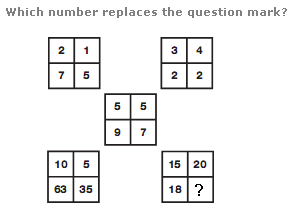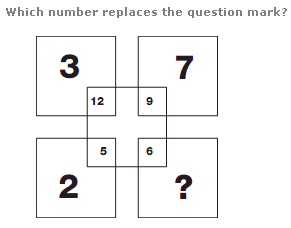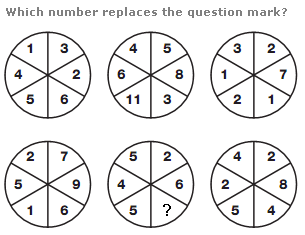# Puzzles - Number puzzles

Exercise : Number puzzles
127.:
14
Explanation
:
Add together numbers in corresponding segments of the upper 2 squares, and put the results in the middle square. Numbers in the lower left square equal the product of the upper left and central squares, and numbers in the lower right square equal the product of the upper right and central squares.

128.:
9
Explanation
:
Taking numbers in diagonally opposite squares, calculate their sum, and put the answer in the small, inner square of the upper number. Calculate their difference, and put this answer in the small, inner square of the lower number.

129.